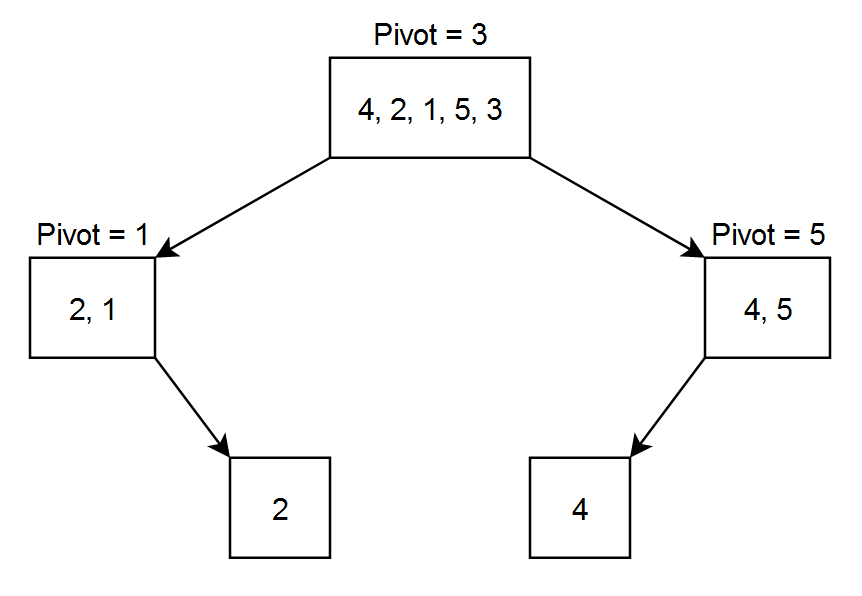# Quick Sort

Posted: 23 Dec, 2020
Difficulty: Moderate

## PROBLEM STATEMENT

#### Example:

``````Let the array = [ 4, 2, 1, 5, 3 ]
Let pivot to be the rightmost number.
``````#### After the 1st level partitioning the array will be { 2, 1, 3, 4, 5 } as 3 was the pivot. After 2nd level partitioning the array will be { 1, 2, 3, 4, 5 } as 1 was the pivot for the left part and 5 was the pivot for the right part. Now our array is sorted and there is no need to divide it again.

##### Input format:
``````The first line of input contains an integer 'T' denoting the number of queries or test cases.

The first line of each input consists of an integer 'N' denoting the size of the array.

The second line of each input consists of 'N' space-separated integers denoting the elements of the array.
``````
##### Output format:
``````For each test case, print a single line containing space-separated integers denoting the elements of the array after sorting.

The output of each test case will be printed in a separate line.
``````
##### Note:
``````You do not need to print anything, it has already been taken care of. Just implement the given function.
``````
``````Can you solve this in the worst case NlogN complexity?
``````
##### Constraints:
``````1 <= T <= 10
1 <= N <= 10 ^ 3
-10 ^ 9 <= ARR[i] <= 10 ^ 9

Where 'T' is the number of test cases, 'N' is the length of the array 'ARR', and 'ARR[i]' is the array element at index i.

Time limit: 1 sec.
``````Approach 1

The first approach will be picking edge elements as pivot.

What needs to be done:

• Pick the rightmost element as pivot.
• Partition the array with numbers smaller than pivot on the left of pivot and numbers larger than pivot on the right of the pivot.
• Recursively sort both left and right partition.